# CNN可视化Convolutional Features

• A+

## 简介

• 输入一堆图片，找出其中一张，使得某个神经元激活最大
• 随机给一张图片，通过梯度下降，修改这张图片的像素，是某个神经元的激活最大

### 关于filter的激活值

• 如现在filter的输出为11*11的一个矩阵（经过一个卷积层的输出）
• 下图a_ij_k表示第k个filter的第i行j列；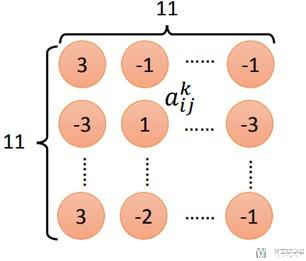• 对输出值进行求和(这里的定义可以根据需要进行改变)；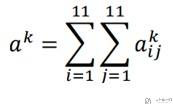• 最终的目标是使得a_k最大；
• 最终，使用梯度下降法进行求解；

## 方法简单介绍

1. 初始化一张图片, 56*56
2. 使用预训练好的VGG16网络，固定网络参数
3. 若想可视化第40层layer的第k个filter的conv, 我们设置loss函数为 (-1*神经元激活值)；
4. 梯度下降, 对初始图片进行更新；
5. 对得到的图片*1.2, 得到新的图片，重复上面的步骤；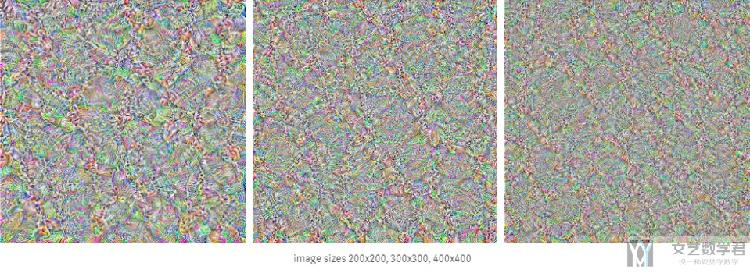## 简单分析

### 随着卷积层的加深, pattern在变得复杂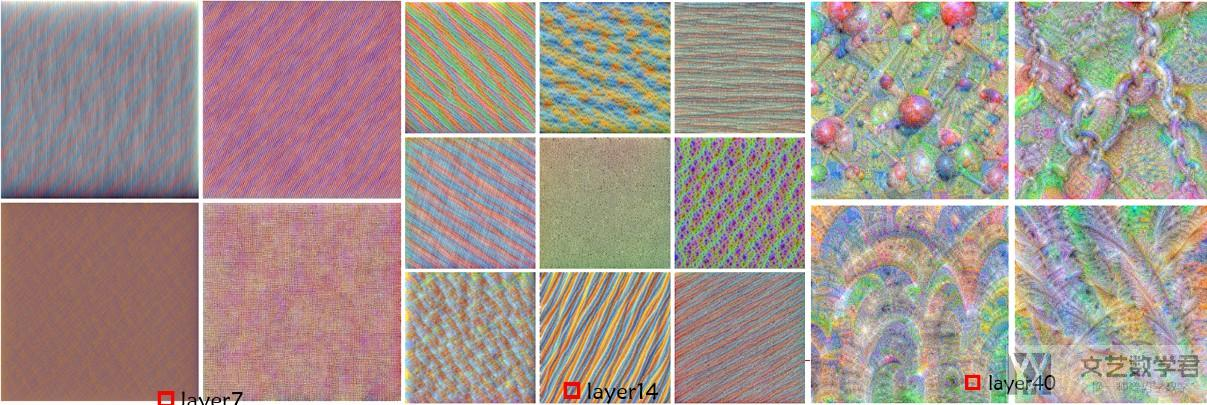### 验证猜想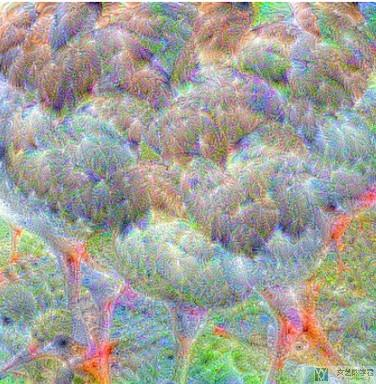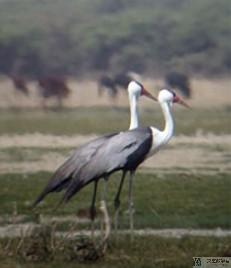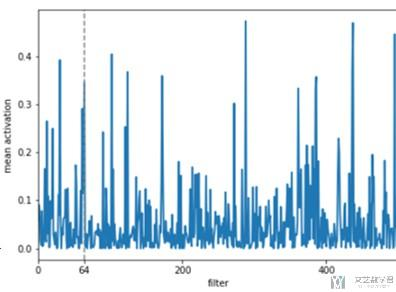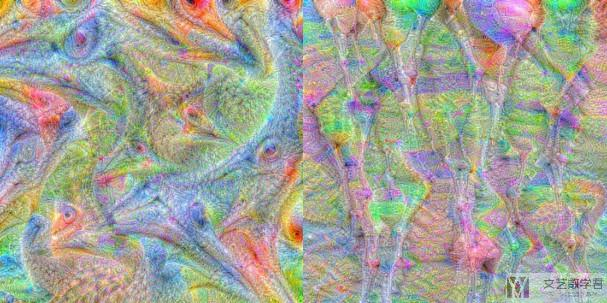Regarding the bottom row, however, I have no clue. Maybe those patterns are associated with the background of the image or simply represent something the network needs for detecting birds that I don’t understand. I suppose this will remain part of the black box for now…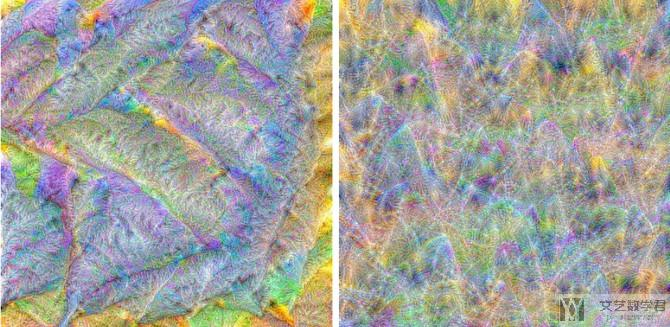## 代码实现

### 导入相应的库

1. import torch
2. from torch.autograd import Variable
3. from PIL import Image,ImageOps
4. import torchvision.transforms as transforms
5. import torchvision.models as models
6. import numpy as np
7. import cv2
8. from cv2 import resize
9. from matplotlib import pyplot as plt
10. %matplotlib inline

1. device = torch.device('cuda' if torch.cuda.is_available() else 'cpu')

### 初始化图片

1. # 生成随机的图片
2. sz = 56
3. img = np.uint8(np.random.uniform(150, 180, (3, sz, sz)))/255
4. # img[None]可以增加一个维度
5. img = torch.from_numpy(img[None]).float().to(device)
6. img.shape
7. # torch.Size([1, 3, 56, 56])

### 导入预训练好的网络

1. model_vgg16 = models.vgg16_bn(pretrained=True).features.to(device).eval()

### 使用hook类

1. class SaveFeatures():
2.     """注册hook和移除hook
3.     """
4.     def __init__(self, module):
5.         self.hook = module.register_forward_hook(self.hook_fn)
6.     def hook_fn(self, module, input, output):
7.         # self.features = output.clone().detach().requires_grad_(True)
8.         self.features = output.clone()
9.     def close(self):
10.         self.hook.remove()

1. layer = 40
2. activations = SaveFeatures(list(model_vgg16.children())[layer])

### 进行反向传播

1. # 超参数
2. lr = 0.1 # 学习率
3. opt_steps = 25 # 迭代次数
4. filters = 265 # 第265个filter,使其最大
5. upscaling_steps = 13 # 图像放大次数
6. blur=3
7. upscaling_factor=1.2 # 把图像变粗

1. # 定义处理时的均值与方差
2. cnn_normalization_mean = torch.tensor([0.485, 0.456, 0.406]).view(-1, 1, 1).to(device)
3. cnn_normalization_std = torch.tensor([0.229, 0.224, 0.225]).view(-1, 1, 1).to(device)

1. for epoch in range(upscaling_steps):  # scale the image up upscaling_steps times
2.     # --------------------------------------------------------------------------------
3.     # 因为原始的VGG网络对图片做了normalization, 所以这里对输入图片也要做normalization
4.     # --------------------------------------------------------------------------------
5.     img = (img - cnn_normalization_mean) / cnn_normalization_std
6.     img
=1
7.     img
=0
8.     print('Imgshape1 : ',img.shape)
9.     img_var = Variable(img, requires_grad=True)  # convert image to Variable that requires grad
10.     # ----------
11.     # 定义优化器
12.     # ----------
13.     optimizer = torch.optim.Adam([img_var], lr=lr, weight_decay=1e-6)
14.     for n in range(opt_steps):  # optimize pixel values for opt_steps times
15.         optimizer.zero_grad()
16.         model_vgg16(img_var) # 正向传播
17.         loss = -activations.features[0, filters].mean() # loss相当于最大该层的激活的值
18.         loss.backward()
19.         optimizer.step()
20.     # ------------
21.     # 图像进行还原
22.     # ------------
23.     print('Loss:',loss.cpu().detach().numpy())
24.     img = img_var * cnn_normalization_std + cnn_normalization_mean # 这个使用img_var变换img
25.     img
=1
26.     img
=0
27.     img = img.data.cpu().numpy().transpose(1,2,0)
28.     sz = int(upscaling_factor * sz)  # calculate new image size
29.     img = cv2.resize(img, (sz, sz), interpolation = cv2.INTER_CUBIC)  # scale image up
30.     if blur is not None: img = cv2.blur(img,(blur,blur))  # blur image to reduce high frequency patterns
31.     print('Imgshape2 : ',img.shape)
32.     img = torch.from_numpy(img.transpose(2,0,1)[None]).to(device)
33.     print('Imgshape3 : ',img.shape)
34.     print(str(epoch),',Finished')
35.     print('=======')
36. activations.close() # 移除hook

1. # 保存图片
2. image = img.cpu().clone()
3. image = image.squeeze(0)
4. unloader = transforms.ToPILImage()
5. image = unloader(image)
6. image = cv2.cvtColor(np.asarray(image),cv2.COLOR_RGB2BGR)
7. cv2.imwrite('res1.jpg',image)
8. torch.cuda.empty_cache()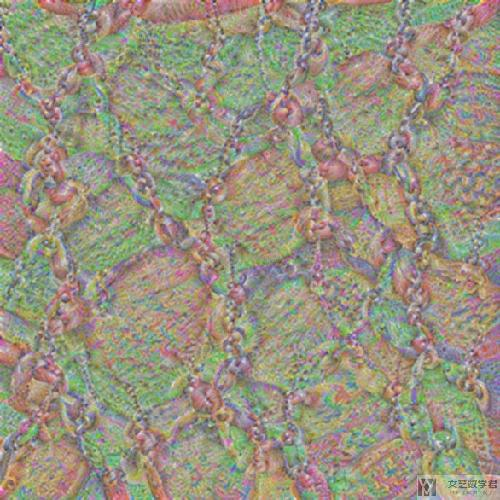### 验证结果

1. size = (224, 224)
2. picture = Image.open("./Broad_chain_closeup.jpg")
3. # picture = Image.open("./Wattledcranethumb.jpg")
4. picture = ImageOps.fit(picture, size, Image.ANTIALIAS)
5. picture1. # 转换为tensor
2. loader = transforms.ToTensor()
3. picture = loader(picture).to(device)
4. # 将图片做标准化, 转换为vgg网络的输入
5. cnn_normalization_mean = torch.tensor([0.485, 0.456, 0.406]).view(-1, 1, 1).to(device)
6. cnn_normalization_std = torch.tensor([0.229, 0.224, 0.225]).view(-1, 1, 1).to(device)
7. # 减均值. 除方差
8. picture = (picture - cnn_normalization_mean) / cnn_normalization_std
9. # 查看转换之后的效果
10. # unload = transforms.ToPILImage()
11. # unload(picture.cpu())

1. # 定义网络
2. model_vgg16 = models.vgg16_bn(pretrained=True).features.to(device).eval()
3. # 对网络进行hook,hook住指定层的output的值
4. layer = 42 # 这里是ReLU之后, 经过激活之后也应该较大
5. filters = 265
6. activations = SaveFeatures(list(model_vgg16.children())[layer])
7. # 网络前向传播
8. with torch.no_grad():
9.     picture_var = Variable(picture[None])
10.     model_vgg16(picture_var)
11. activations.close() # 移除hook

1. # 画出每个filter的平均值
2. mean_act = [activations.features[0,i].mean().item() for i in range(512)] # 计算平均值
3. plt.figure(figsize=(7,5))
4. act = plt.plot(mean_act,linewidth=2.) # 画出折线图
5. extraticks=[filters] # 增加的一个坐标
6. ax = act.axes
7. ax.set_xlim(0,500)
8. plt.axvline(x=filters, color='grey', linestyle='--') # 绘制虚线
9. ax.set_xlabel("feature map")
10. ax.set_ylabel("mean activation")
11. ax.set_xticks([0,200,400] + extraticks)
12. plt.show()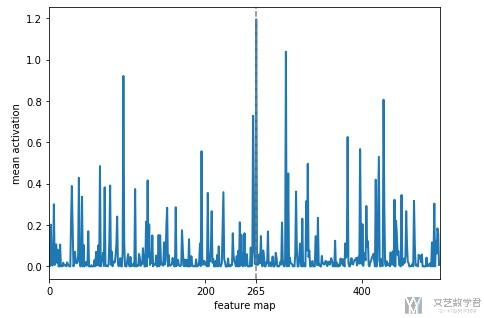## 结语

CNN可视化的一些解释-Github仓库地址

• 微信公众号
• 关注微信公众号
•• QQ群
• 我们的QQ群号
•